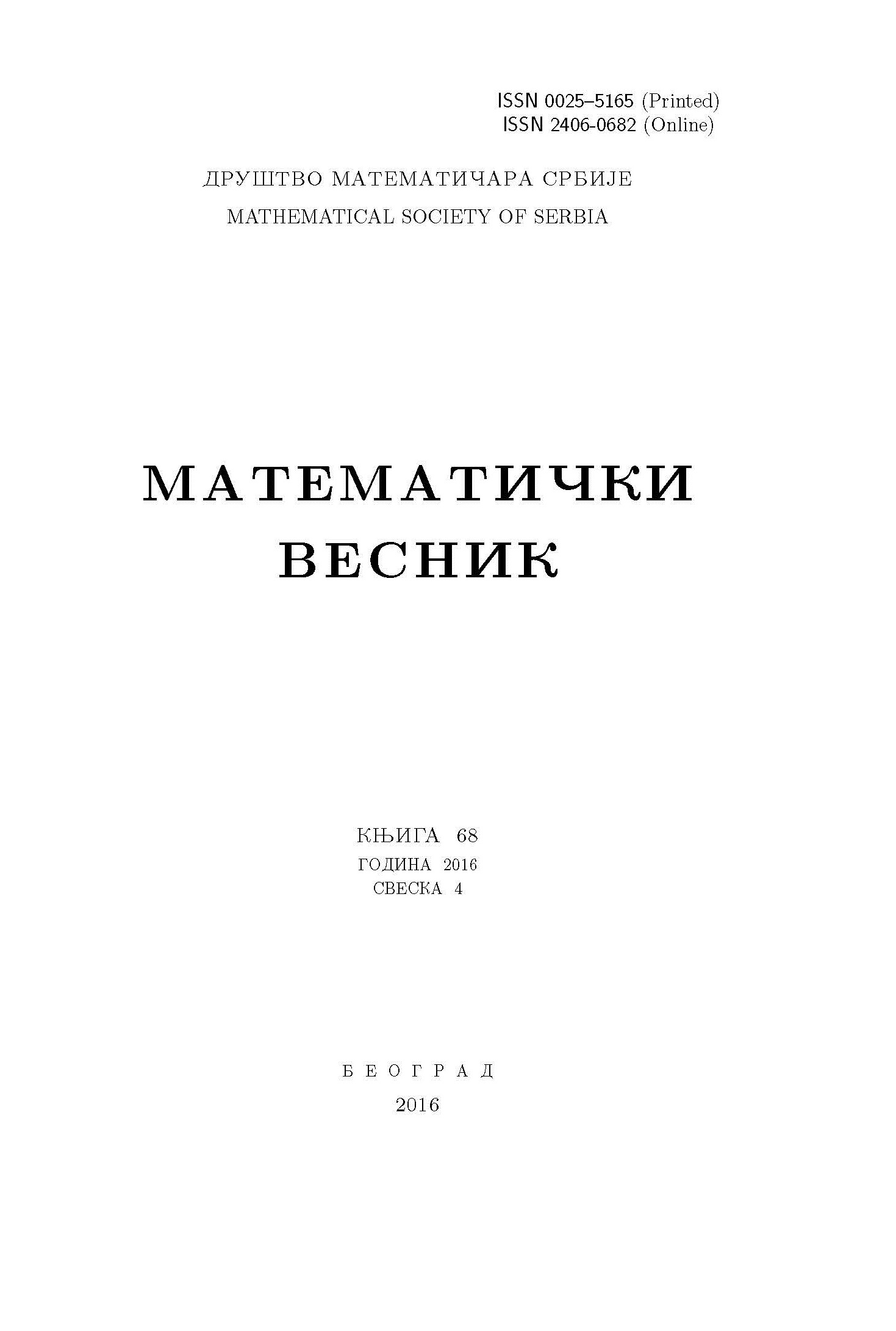﻿ Matematički Vesnik ﻿
 MATEMATIČKI VESNIK МАТЕМАТИЧКИ ВЕСНИКNAPOLEON'S THEOREM FROM THE VIEW POINT OF GRÖBNER BASES M. Čvorak, M. Muzika Dizdarević AbstractIn this article, we present a new proof of the Napoleon's theorem using algorithmic commutative algebra and algebraic geometry. We also show that, by using the same technique, several related theorems, with the same basic set of objects can be proved. Thus, from the new proof of Napoleon's theorem, we prove the Relative of Napoleon's theorem (result given by B. Grünbaum). Then, we present a new theorem related to Napoleon's theorem. In this theorem the existence of two more quadruplets of equilateral triangles associated with a given triangle was established.Keywords: Napoleon's theorem; automatic theorem proving; elementary geometry; Gröbner bases. MSC: 13P10, 68T15, 51M04 Pages:  289$-$300 Volume  74 ,  Issue  4 ,  2022

﻿